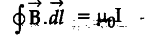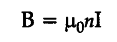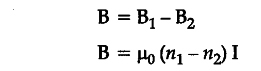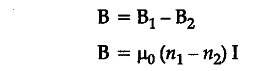# State Ampere's circuital law, expressing it in the integral form

(a) State Ampere’s circuital law, expressing it in the integral form.
(b) Two long co-axial insulated solenoids, S1 and S2 of equal lengths are wound one over the other as „ shown in the figure. A steady current “I” flows through the inner solenoid S1 to the other end B, which is connected to the other solenoid S2 through which the same current “I” flows in the opposite direction so as to come out at end A. If n1 and n2 are the number of turns per unit length, find the magnitude and direction of the net magnetic field at a point (i) inside on the axis and (ii) outside the combined system.(a) Ampere’s circuital law : The line integral of the magnetic field around a closed path is times of total current enclosed by the path.Expression of the law in integral form :(b) (i)Magnitude of net magnetic field inside the combined system on the axis,Also accept if the student writes(ii) Outside the combined system, the net magnetic field is zero.